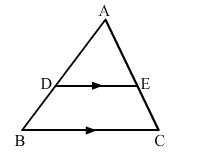# In ∆ABC, DE is drawn parallel to BC, cutting AB and AC at D and E, respectively, such that AB = 7.2 cm

Question:

In ∆ABCDE is drawn parallel to BC, cutting AB and AC at and E, respectively, such that AB = 7.2 cm, AC = 6.4 cm and AD = 4.5 cm. Find AE.(a) 5.4 cm
(b) 4 cm
(c) 3.6 cm
(d) 3.2 cm

Solution:

(b) 4 cm

It is given that DE">BC.

Applying basic proportionality theorem, we get:

$\frac{A D}{A B}=\frac{A E}{A C}$

$\Rightarrow \frac{4.5}{7.2}=\frac{A E}{6.4}$

$\Rightarrow A E=\frac{4.5 \times 6.4}{7.2}=4 \mathrm{~cm}$